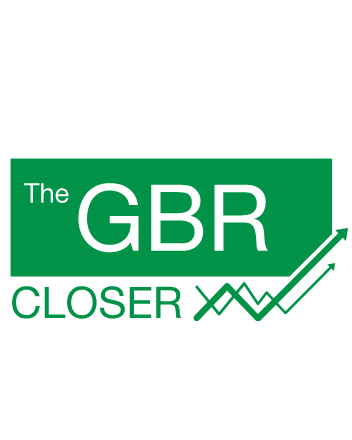# How to Calculate ROI

Understand how return on investment works.

Any time you invest — whether it’s in stocks, bonds, real estate or your own business — you want to measure how well the investment is performing. Although you can calculate your profit or loss using raw numbers, that calculation doesn’t take into consideration the amount you had to invest to generate those returns.

For example, if a stock earns you a \$100 return, that’s certainly better than a loss. However, the return on investment, or ROI, would be much more impressive if you had only invested \$500 rather than investing \$5,000.

Whether you’ve been investing for a long time, or are turning your tax refund into an investment for the first time, read on to learn how to use the ROI formula.

## ROI Definition

You can find an ROI calculator online, but understanding how the formula works helps you better understand your investment returns. ROI measures the return your investment has generated relative to the amount invested. So, instead of giving you a dollar amount for how much you’ve made or lost, your ROI is measured as a percentage of the total you invested. When calculating your ROI, make sure you include all the profits and expenses. For example, if you bought a stock and held it for five years, include the value of the dividends you received during that time period.

Building Wealth

Choose Wisely: The Best Online Stock Brokers for Beginners

## How to Calculate ROI

You don’t need a return on investment calculator to measure your returns with the ROI formula, just the amount you initially invested and what the value of the investment has grown to.

• First, subtract the initial investment from the current value to figure your gain or loss on your investment.
• Second, divide the gain or loss by your initial investment to figure the ROI as a rate.
• Third, multiply the ROI as a rate by 100 to convert it to a percentage.

ROI = [(Current value – initial investment)/initial investment] x 100

For example, say you invested \$800 in the stock market and the value of your portfolio has grown to \$1,000.

• First, subtract \$800 from \$1,000 to find you have a \$200 profit.
• Second, divide \$200 by \$800 to find your ROI as a rate is 0.25.
• Third, multiply 0.25 by 100 to find your ROI on your investments is 25 percent.

If you have a loss, the formula works the same way, but you will end up with a negative ROI. For example, say your portfolio didn’t do so well and dropped from \$800 to \$600.

• First, subtract \$800 from \$600 to find you have a \$200 loss.
• Second, divide negative \$200 by \$800 to find your ROI as a rate is negative 0.25.
• Third, multiply negative 0.25 by 100 to find your ROI is negative 25 percent.

More on Investing

#### Michael Keenan

Michael Keenan is a writer based in the Kansas City area, specializing in personal finance, taxation, and business topics. He has been writing since 2009 and has been published by Quicken, TurboTax and The Motley Fool.## Sending you timely financial stories that you can bank on.